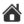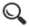﻿ Introduction to arrlib
 Up | Introduction to arrlib | arrlib versus arr/geom | arrlib examples | Complete arrlib function list | Using Numpy with Scorpion VisionIntroduction to arrlib
Small elements

You can create singletons of small vectors, matrices and special elements with arrlib. You can easily construct such elements and manipulate them, including setting and getting subelements, using them as parameters to arrlib functions, and performing arithmetic:

Small elements, general

 Vectors xyf xyzf xyzwf xyzwvf xyd xyzd xyzwd xyzwvd xyi xyzi xyzwi v = arrlib.xyf() 2-dim float element v = arrlib.xyf(10,20) 2-dim float element with initial value v = arrlib.xyzd() 3-dim double element v = arrlib.XYi(1,2) 2-dim integer element with initial value Matrices m22f m33f m43f m44f m22d m33d m43d m44d m = arrlib.m22f() 2x2 float matrix m = arrlib.m33d((1,0,0),(0,1,0),(0,0,1)) 3x3 double matrix with initial value Lines lin2f lin3f lin2d lin3d l = arrlib.lin2d() 2dim double line l = arrlib.lin2f((0,0),(10,5)) 2dim float line with (point),(direction) value Boxes box1f box2f box3f box1d box2d box3d box1i box2i box3i b = arrlib.box2i((0,0),(10,10)) 2dim integer box with (min),(max) value b = arrlib.box1f(0.0,10.0) 1dim float box with min,max value Circles cir2f cir3f cir2d cir3d c = cir2f((10,10),5) circle with center=(10,10) and radius=5 c = cir3d((0,0,0),10) sphere at origin with radius=10

Special small elements

 Matrices identf rotf xlatf scalf identd rotd xlatd scald i = arrlib.identf(3) 3x3 float identity matrix r = arrlib.rotf(1.2) 3x3 rotation matrix - 1.2 radians around z axis (3x3 matrix) r = arrlib.rotf(1.0,0,0) 3x3 rotation matrix - 1.0 radians around x axis (3x3 matrix) s = arrlib.xlatf(2,2) homogenous 2dim translation (3x3 matrix) s = arrlib.scald(1,2,3) homogenous 3dim scale factor (4x4 matrix)

Subelement access

 Vectors x y z w v v.x = 10 element assignment e = v.w element extraction Matrices m11 ... m44 m.m33 = 1 element assignment e = m.m22 element extraction Lines p v l.p = 10,10 tuple assignment to 2dim start point l.v = 1,0,0 tuple assignment to 3dim direction Boxes min max b.min = 10,10 tuple assignment to 2dim min max = b.max tuple extraction of max Circles c r c.c = 5,5 center assignment c.r = 10 radius assignment

Large vectors and matrices

arrlib supports large vectors and matrices of scalars as well as all the small elements. This is useful for representing e.g. images, polygons, resample grids etc. Subelements can be accessed as tuples. Most natural mathematical operations are allowed - see below.

 Vectors floatVec doubleVec intVec uintVec uint8Vec xyfVec xyzfVec xyzwfVec xyzwvfVec xydVec xyzdVec xyzwdVec xyzwvdVec xyiVec xyziVec xyzwiVec m22fVec m33fVec m43fVec m44fVec m22dVec m33dVec m43dVec m44dVec V = arrlib.xyfVec(100) 100-element 2dim polygon V = 10,20 tuple assignment to subelement x,y = V subelement extraction Matrices floatMat doubleMat intMat uintMat uint8Mat xyfMat xyzfMat xyzwfMat xyzwvfMat xydMat xyzdMat xyzwdMat xyzwvdMat xyiMat xyziMat xyzwiMat m22fMat m33fMat m43fMat m44fMat m22dMat m33dMat m43dMat m44dMat im = arrlib.uint8Mat(100,100) 100x100 grayscale image im = 100 only 1-dimensional element access supported M = floatMat(7,7) 7x7 float matrix Special matrices identfMat identdMat I = identfMat(7) 7x7 float identity matrix

Geometric operations

A small matrix can (post-)multiply small vectors, matrices and lines, and large vectors og matrices of "correct" type from arrlib. When using large vectors or matrices, each element in the vector or matrix is then multiplied.

If a large arrlib object contains xyf or xyd elements, postmultiplying by a 3x3 small matrix will automatically trigger a homogenous operation. This means each element in the large object is treated as a 1x3 row vector [x y 1], and a matrix multiplication is performed, resulting in a new set of 1x3 vectors. These are converted back to 2-dim rectangular coordinates, and the result is a new xyf or xyd PyArr object. The same goes for xyzf elements and 4x4 small matrices.

Note that this is very different from e.g. multiplying large float-matrices. Large matrices of types float or double can be multiplied, using normal matrix multiplication rules.

Dividing by a small matrix is the same as multiplying by the inverse. You can add and subtract small vectors or matrices directly using + og -. abs() gives the determinant of a small matrix, and ~ the inverse. A scalar can also be added to, subtracted from, multiplied into or divided into a small vector or matrix. Multiplying two small vectors gives the dot product. .T transposes a matrix. .f, .d or .i converts to float, double or integer, respectively.

Examples:

 V = arrlib.xyfVec(100) 100-element 2dim polygon M = arrlib.xyzfMat(10,10) 10x10 matrix of 3dim points fm = arrlib.floatMat(6,6) 6x6 float matrix im = arrlib.uint8Mat(100,100) grayscale image x = arrlib.xlatf(1,1) 3x3 homogenous translation matrix r = arrlib.rotf(0.1,0.2,0.3) 3x3 rotation matrix m = arrlib.m33f((1,2,3),(-1,0,7),(0,0,1)) a 3x3 matrix v = arrlib.xyf(1,2) a small vector V = V * x translate each 2dim vector element M = M / r inverse rotation of all 3dim points in the matrix fm = fm * fm normal matrix multiplication x2 = x * x 3x3 translation matrix (translates twice as far) m = ~m inverse of m m = m.T transpose of m m = m.d m converted to double d = v * v dot product (a scalar) m = m * 2 elementwise multiplication v = v / 2.4 elementwise division

Assignment

You can assign to subelements in a small variable as given in the sections above. Note also that the subelement ".data" always refers to a tuple containg all subelements. For example:

 v = arrlib.xyzf() all subelements contain zero v.data = 1,2,3 same as v = arrlib.xyzf(1,2,3)

More about large vectors

 x = arrlib.xyfVec(10,'tag') Optional tag to name the object x.tag = 'NewFloatVec' New tag assignment z = x.copy() Copying (tag included) z.dim() Vector dimension (number of elements) z.elemtype() 'float', 'int', 'XYf' etc... z.isvec() returns true w = z.subVec(start,len) Copy of subvector

More about large matrices

 M = arr.doubleMat(10,10,'matrix') optional tag M.ismat() returns true M.transposeMatr() transpose M.flipUpDown() flip M.flipLeftRight() flip M.rotateMatr(1) rotate 90 degrees M.rowVec(0) extract row 0 M.colVec(0) extract column 0

Field extraction (large vectors and matrices)

You can extract subelements from a large vector or matrix:

• v = arr.xyfVec(10)
• x = v.extractfield('x')

Return value is another large object. The allowed field names are as follows:

 xyf xyd xyi 'x' 'y' xyzf xyzd xyzi 'x' 'y' 'z' xyzwf xyzwd xyzwi 'x' 'y' 'z' 'w' xyzwvf xyzwvd 'x' 'y' 'z' 'w' 'v' lin2f lin3f lin2d lin3d 'p' 'v' box1f box1d box1i .. box3f box3d box3i 'min' 'max' cir2f cir2d cir3f cir3d 'c' 'r' m22f .. m44d 'm11' ..'m44' rgb rgbf 'r' 'g' 'b' hsi hsif 'h' 's' 'i'

Alignment

To support SSEx routines, vectors and matrices can be aligned to specific addresses in memory. An extra parameter is used in a call to the constructor function:

• x = arrlib.Vec('XYf',10,'tag',4)
• m = arrlib.Mat('uint8',10,16,'floatmat',4)

Here, data is aligned to 1<<4 bytes (16). For matrices, this is only allowed when each row will be similarly aligned -- in the latter case above, the number of columns must be a multiple of 16 (since bytes -- uint8 -- are being allocated).

Trees

A Tree is a vector of pointers. Each element addresses another large object. Trees can be nested.

• t = arrlib.Tree('Tree')
• t = arrlib.Tree('Tree',10)
• Tag and dimension kan be omitted
• No dimension means an empty tree
• If dimension is specified, the result is a tree with the given number of empty "branches"
• t.istree() returns true
• Assigns child to an empty spot in t. The tree is expanded if necessary
• Tag can be omitted -- if omitted and the new child element already has a tag, it is used.
• t = m
• Assignment to given position, any existing child at the given position is removed
• Elements may only be child in one tree at the time. Attempts to assign a child into multiple trees result in failure
• t.intvec
• Access to child element via tag name
• t
• Similar, but access per position
• del t
• t = None
• Removes child element. (Note: "del t.intvec" or "t.intvec = None" is not supported.)
• t.unhook('intvec')
• Removes child from the tree, via tag name. If there are no further Python references to the element, it is deleted altogether..
• t2 = t.copy()
• Deep copy of the tree structure, including tag names.

Blobs

• Realised as a separate type "blob"
• property: area
• property: nholes
• property: nchunks
• property: box
• Blobarray is realised in Python as a tuple of this type
• Constructed only as return value of special functions, no general constructor

Blob functions exported from ArrLib

• polygonBlob
• findBlobs
• colorBlobs
• findBlobsInBlob
• erodeBlobs
• dilateBlobs
• skeletBlobs
• blobContour
• blobPolygon
• blobCenterOfGravity
• blobIntersection
• blobSubtraction
• blobUnion
• decomposeBlob

See the Blob section under examples for more information.

Functions exported from ArrLib (arrlib.<func>):

Most functions in ArrLib are exported via arrlib, some also as member functions to large objects.

These functions are NOT exported:

• Chunk-related functions (chunks are not supported).
• Many functions operating on "fixed" numbers (in general, "fixed" is not at all supported in arrlib).
• Note that you can very well use e.g. arrlib.toFix(), but you cannot index the result -- the result must be used as input to another function.

 Scorpion Vision Version XII : Build 646 - Date: 20170225 Scorpion Vision Software® is a registered trademark of Tordivel AS. Copyright © 2000 - 2017 Tordivel AS.